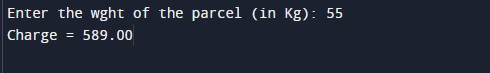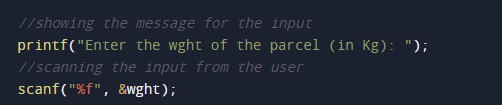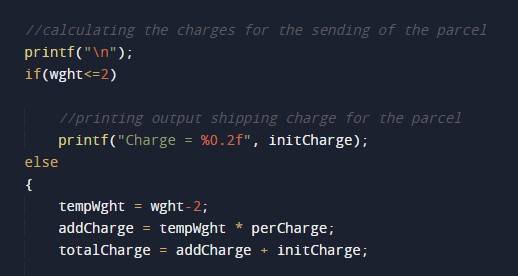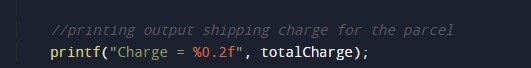C Program to calculate Charges for Sending Parcels as per Weight

In this tutorial you will learn about the C Program to calculate Charges for Sending Parcels as per Weight and its application with practical example.

C Program to calculate Charges for Sending Parcels as per Weight

In this tutorial, we will learn to create a C program that will Charge for Sending Parcels as per Weight using C programming.

Charges for Sending Parcels as per Weight

Prerequisites

Before starting with this tutorial we assume that you are best aware of the following C programming topics:

• Operators in C Programming.
• Basic Input and Output function in C Programming.
• Basic C programming.
• While loop in C programming.
• Arithmetic operations in C Programming.

Program to Charges for Sending Parcels as per Weight

In c programming, it is possible to take numerical input from the user and Charges for Sending Parcels as per Weight with the help of a very small amount of code. The C language has many types of header libraries which has supported function in them with the help of these files the programming is easy. But today we will Charge for Sending Parcels as per Weight using c programming.

As per the following rule:

The minimum charge for a parcel equal to or lighter than the 2k.g. will be 32.50 and for each extra Kg,  there is an additional charge of 10.50

Algorithm:-

With the help of this program, we can Charge for Sending Parcels as per Weight.

Output:-In the above program, we have first initialized the required variable.• wght= it will hold the floating value.
• initCharge = it will hold the floating value initial value 32.50.
• perCharge = it will hold the floating value initialvalue 10.50.
• tempWght= it will hold the floating value.
• addCharge= it will hold the floating value.
• totalCharge= it will hold the floating value.

Input weight for the parcel.Program Logic Code.Printing output for shipping calculated.In this tutorial we have learn about the C Program to calculate Charges for Sending Parcels as per Weight and its application with practical example. I hope you will like this tutorial.